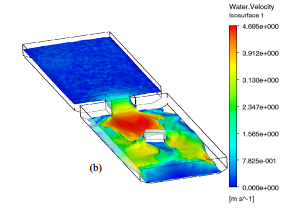# Using the Fast Multi-Objective Genetic Algorithm to Improve the Urban Flood Modeling

Author(s):
M. Rezoug, R. E. Meouche, R. Hamzaoui, and Z.-Q. Feng (IACSIT International Journal of Engineering and Technology)

CFD modeling Iso-surface of water height colored by the velocity magnitude at t=8s of the simulation. In the present paper, a multi objective optimization approach based in Genetic Algorithm, Design Of Experiments and Response Surface Method strategies, is applied in order to predict the optimal CFD model parameters, allowing to model with high accuracy the 3D free surface flow of urban flood propagation retaining the total computation time (CPU-time) as short as possible. An experimental data set was used in this study as a validation means for numerical optimized models. We can say that the constraints in terms of computation time and accuracy, related to the application of 3D CFD modeling for flood propagation problems can be overcome by making an optimal choice of the advanced parameters of model.